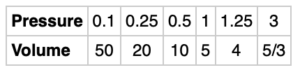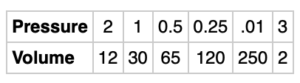# Volume and Pressure

Boyle’s law is an important law in the physical sciences, it states that for a given set of conditions, the pressure and volume of a gas are inversely proportional. For a specific gas, a scientist made the following pairs of measurements:Inversely proportional relationships have a constant of proportionality. What is the constant of proportionality for this gas? How did you arrive at this constant of proportionality and can you generalize it? How does this relate to the graph of the data?How do you know that the scientist made an error in the measurements of this gas?